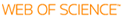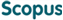### BROWSE

#### Related ResearcherSong, Hyun-Kon
eclat: ElectroChemistry Lab of Advanced Technology
Research Interests
• Electrochemical analysis, electroactive materials, electrochemistry-based energy devices

Electrochemical porosimetry: Deconvolution of distribution functions

Cited 4 times inCited 5 times inTitle
Electrochemical porosimetry: Deconvolution of distribution functions
Author
Keywords
Deconvolution; Distribution function; Fourier transform; Impedance; Low-pass filtering
Issue Date
2006-07
Publisher
ELSEVIER SCIENCE INC
Citation
ELECTROCHEMISTRY COMMUNICATIONS, v.8, no.7, pp.1191 - 1196
Abstract
Discrete Fourier transform (DFT) was used to deconvolute the distribution of penetrability coefficient (alpha(0)-distribution, equivalent to pore size distribution) from electrochemical impedance data of porous electrodes. The working equation is the Fredholm. integral equation of the first kind to correlate macroscopic impedance data to a theoretical model describing microscopic signal with the alpha(0)-distribution. Simulated and experimental impedance data were tested. Noise observed at high frequencies in Fourier space was removed before inversely Fourier-transforming the alpha(0)-distribution from Fourier space to real space. The accuracy of alpha(0)-distributions deconvoluted by DFT depended on the number, frequency range and quality of impedance data. The examples in this work showed that fairly accurate alpha(0)-distributions could be obtained by DFT deconvolution. Most promising method was to use the alpha(0)-distribution obtained from DFT deconvolution as the first guess to shape the true alpha(0)-distribution. Then, accurate alpha(0)-distributions could be obtained by estimating parameters of the pre-assumed distributions by using complex nonlinear least square fitting.
URI
DOI
10.1016/j.elecom.2006.05.012
ISSN
1388-2481
Appears in Collections:
ECHE_Journal Papers
Files in This Item: